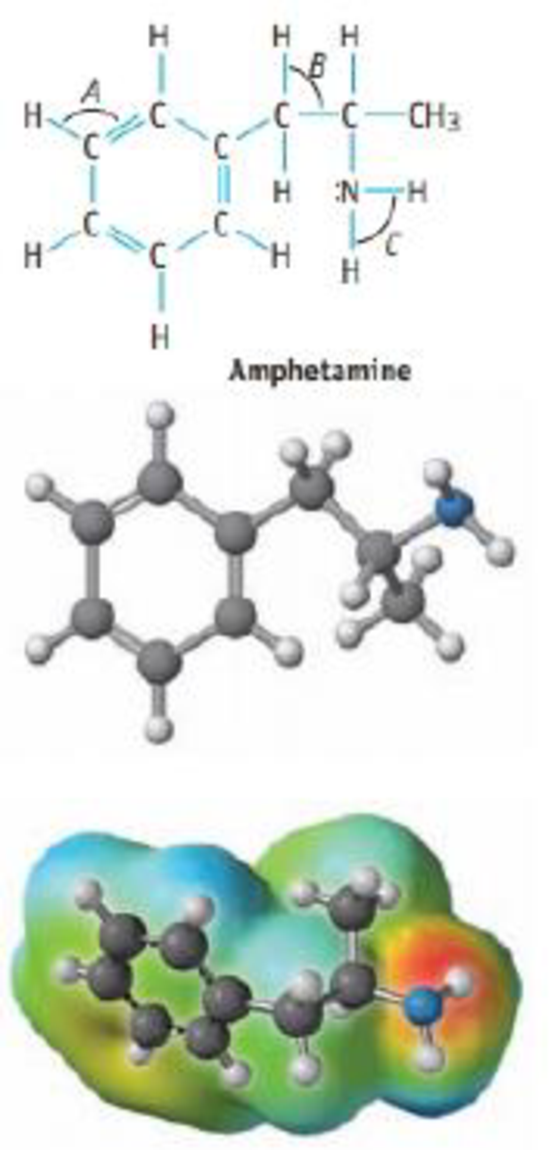Chapter 9, Problem 48GQ

Chapter
Section
Textbook Problem

The structure of amphetamine, a stimulant, is shown below. (Replacing one H atom on the NH2, or amino, group with CH3 gives methamphetamine a particularly dangerous drug commonly known as “speed.”)(a) What are the hybrid orbitals used by the C atoms of the C6 ring. by the C atoms of the side chain, and by the N atom?(b) Give approximate values for the bond angles A, B, and C.(c) How many σ bonds and π bonds are in the molerule?(d) Is the molecule polar or nonpolar?(e) Amphetamine reacts readily with a proton (H+) in aqueous solution. Where does this proton attach to the molecule? Explain how the electrostatic potential map predicts this site of protonation.

(a)

Interpretation Introduction

Interpretation:

The hybrid orbitals used by the carbon atoms of the C6 ring, by the Carbon atom of the side chain and by the nitrogen atom in the given Amphetamine molecule should be determined.

Concept Introduction:

Hybridization:  When the atomic orbitals mix to form new atomic orbitals, the new orbitals have the same total electron capacity as the old ones. The properties and energies of the new, hybridized orbitals are an 'average' of the original unhybridized orbitals.

sp2 hybridization: This concept  has intermixing of one s-orbitals and two p-orbitals to give three identical hybrid orbitals is known as Sp2 or trigonal hybridization".

sp3 hybridization: This concept  has intermixing of one s-orbitals and three p-orbitals to give four identical hybrid orbitals is known as Sp3 or tetragonal hybridization".

Geometry of different types of molecule with respect to the hybridizations are mentioned are mentioned below,

TypeofmoleculeHybridaizationAtomicorbitalsusedforhybridaizationGeometryAX2sp1s+1pLinearAX3,AX2Bsp21s+2pTrigonalplanarAX4,AX3B,AX2B2sp31s+3pTetrahedralAX5,AX4B,AX3B2,AX2B3sp3d1s+3p+1dTrigonalbipyramidalAX6,AX5B,AX4B2sp3d21s+3p+2dOctahedralACentralatomXAtomsbondedtoABNonbondingelectronpairsonA

Explanation

Given structure of Amphetamine molecule is,

The hybridization of amphetamine molecule can be determined as follows,

(b)

Interpretation Introduction

Interpretation:

Approximate values for the bond angles A,B and C in the given molecule should be determined.

Concept Introduction:

Hybridization:  When the atomic orbitals mix to form new atomic orbitals, the new orbitals have the same total electron capacity as the old ones. The properties and energies of the new, hybridized orbitals are an 'average' of the original unhybridized orbitals.

sp2 hybridization: This concept  has intermixing of one s-orbitals and two p-orbitals to give three identical hybrid orbitals is known as Sp2 or trigonal hybridization".

sp3 hybridization: This concept  has intermixing of one s-orbitals and three p-orbitals to give four identical hybrid orbitals is known as Sp3 or tetragonal hybridization".

TypeofmoleculeHybridaizationGeometryBondangleAX2spLinear180°AX3,AX2Bsp2Trigonalplanar120°AX4,AX3B,AX2B2sp3Tetrahedral109.5°AX5,AX4B,AX3B2,AX2B3sp3dTrigonalbipyramidal120°,90°AX6,AX5B,AX4B2sp3d2Octahedral90°ACentralatomXAtomsbondedtoABNonbondingelectronpairsonA

(c)

Interpretation Introduction

Interpretation:

Number of σandπ bonds in the given molecule should be determined.

Concept Introduction:

Sigma (σ) bonds are the bonds in which shared hybrid orbital’s electron density are concentrated along the internuclear axis.

Pi (π) bonds are the bonds in which shared unhybridized orbital’s (p, d, etc) electron density are concentrated in above and below of the plane of the molecule.

TypeofBondNo.ofσbondsNo.ofπbondsSingle10Double11Triple12

(d)

Interpretation Introduction

Interpretation:

Check whether the given molecule is polar or not.

Concept Introduction:

Polar molecule has polar covalent bond which involves an unequal sharing of electrons between two bonded atoms; it creates a partial separation of charge and a dipole moment.

Covalent bonds are polar, if there is a difference in electronegativity between the bonded atoms.

(e)

Interpretation Introduction

Interpretation:

Amphetamine reacts readily with a proton in aqueous solution. It should be determined that where does this proton attach to the molecule and explain how the electrostatic potential maps predicts this site of protonation.

Concept Introduction:

Protonation is the addition of a proton to an atom, molecule, or ion.

Still sussing out bartleby?

Check out a sample textbook solution.

See a sample solution

The Solution to Your Study Problems

Bartleby provides explanations to thousands of textbook problems written by our experts, many with advanced degrees!

Get Started

Find more solutions based on key concepts